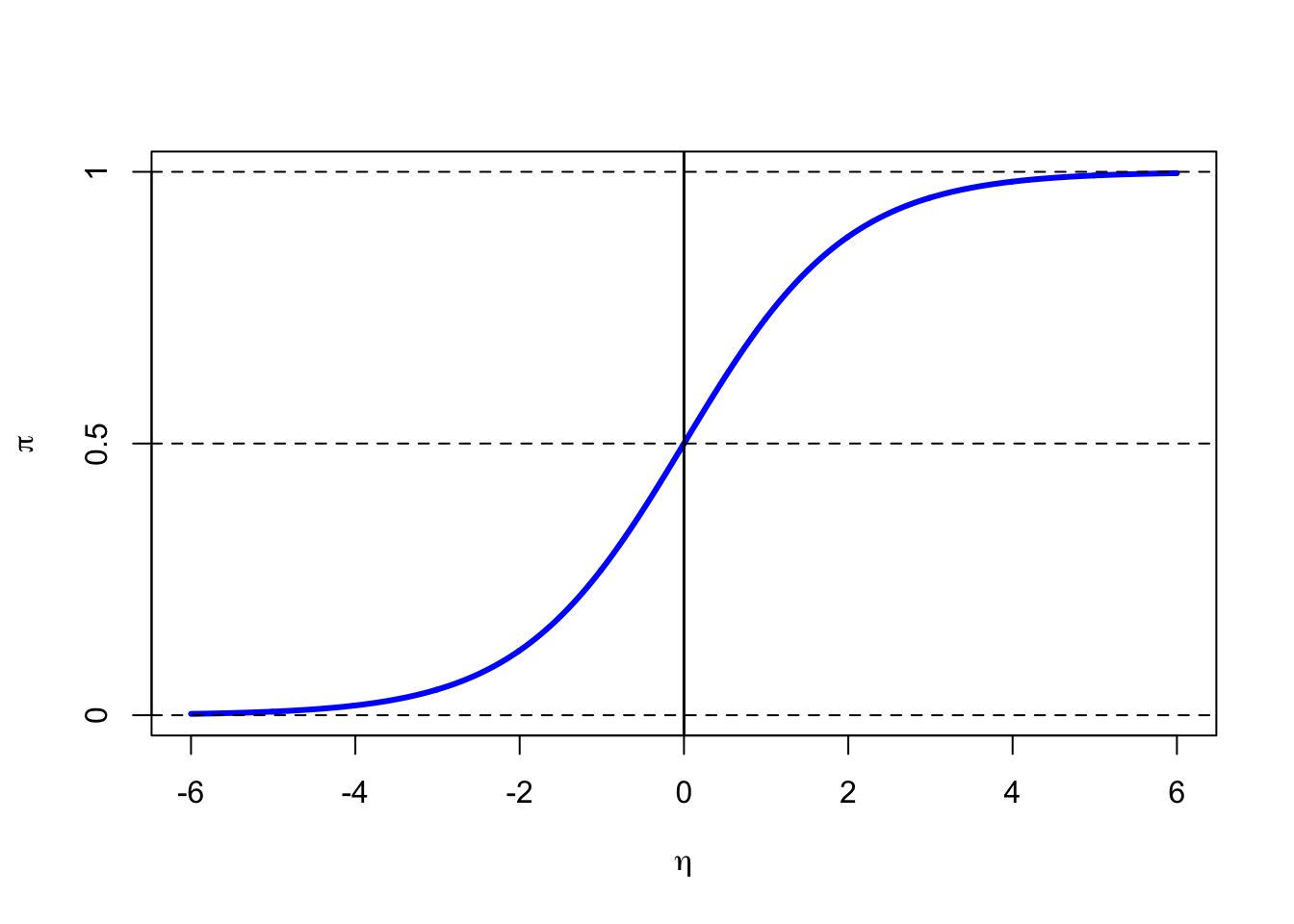The output of a logistic regression is a probability $$(\pi)$$, thus a value between $$0$$ and $$1$$. Moreover, this output is a linear function of known covariates $$x_i$$, which is just another word for the observations in our data set. $\pi =\beta_0+ \beta_1x_1+ \beta_2x_2+ ... +\beta_kx_k$ For a simple logistic regression model with one predictor variable the equation from above simplifies to to

$\pi = \beta_0+ \beta_1x_1\text{.}$ However, the right term of the equation can take any real value, whereas the left term of the equation is a probability, on the scale $$0$$ to $$1$$. In order to transform the scale of the data (right term) into a probability between $$0$$ and $$1$$ we apply a so-called link function.

For the logistic regression model this link function is the logit function. The logit function maps probabilities from the range $$(0, 1)$$ to the entire real number range $$(-\infty, \infty)$$. It is written as

$\eta = logit(\pi)\text{,}$

where $$\pi$$ is the probability.

To understand the logit we first introduce the odds ratio or in short odds. The odds (o) can be written as

$o = \frac{\pi}{1-\pi}\text{,}$

where $$\pi$$ is the probability that an event occurs. If the probability of an event is a $$0.5$$, the odds are one-to-one or even $$\left(\frac{0.5}{1-0.5}=1\right)$$. If the probability is $$1/3$$, the odds are one-to-two $$\left(\frac{1/3}{1-1/3}=1/2\right)$$. The odds can take any positive value and therefore have no ceiling restriction $$[0,\infty)$$. Thus, we further define the or log-odds, which is the logarithm of the odds: $\eta = logit(\pi)= log \left( \frac{\pi}{1-\pi}\right)$

This logarithmic function has the effect of removing the floor restriction, thus the function, the logit function, our link function, transforms values in the range $$0$$ to $$1$$ to values over the entire real number range $$(-\infty, \infty)$$. If the probability is $$1/2$$ the odds are even and the logit is zero. Negative logits represent probabilities below one half and positive logits correspond to probabilities above one half.

The inverse form of the logit function is also called the logistic function, sometimes simply abbreviated as sigmoid function due to its characteristic S-shape. Is allows us to go back from logits to probabilities.

$\pi =logit^{-1}(\eta)= \frac{e^{\eta}}{1+e^{\eta}}=\frac{1}{1+e^{-\eta}}=\frac{1}{1+e^{-\beta_0+ \beta_1x_1+ \beta_2x_2+ ... +\beta_kx_k}}$

The logistic function for the interval $$[-6,6]$$ is shown below. For values of $$\eta$$ in the range from $$-\infty$$ to $$\infty$$ $$\pi$$ is in the range of $$0$$ to $$1$$.